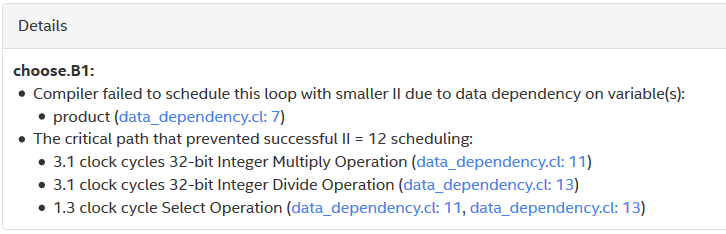ID 683521
Date 12/19/2022
Public

## 3.4.2. Loop-Carried Dependencies that Affect the Initiation Interval of a Loop

There are cases where a loop is pipelined but it does not achieve an II value of 1. These cases are usually caused by data dependencies or memory dependencies within a loop.

### Data Dependencies

Data dependency refers to a situation where a loop iteration uses variables that rely on the previous iteration. In this case, a loop can be pipelined, but its II value may be greater than 1. Consider the following example:

 1  // An example that shows data dependency
2  // choose(n, k) = n! / (k! * (n-k)!)
3
4  kernel void choose( unsigned n, unsigned k,
5                      global unsigned* restrict result )
6  {
7      unsigned product = 1;
8      unsigned j = 1;
9
10      for( unsigned i = k; i <= n; i++ ) {
11          product *= i;
12          if( j <= n-k ) {
13              product /= j;
14          }
15          j++;
16      }
17
18      *result = product;
19  }

For every loop iteration, the value for the product variable in the kernel choose is calculated by multiplying the current value of index i by the value of product from the previous iteration. As a result, a new iteration of the loop cannot launch until the current iteration finishes processing.

The loop in kernel choose has an II value of 12. This information can be found in the Loop Analysis report. In addition, the details pane in the following figure shows that the high II value is caused by a data dependency on product, and the largest contributor to the critical path is the integer division operation on line 13.

Figure 56. Details Pane of the Loop Analysis Report for the Kernel choose### Memory Dependency

Memory dependency refers to a situation where memory access in a loop iteration cannot proceed until memory access from the previous loop iteration is completed. Consider the following example:

1  kernel void mirror_content( unsigned max_i,
2                              global int* restrict out)
3  {
4    for (int i = 1; i < max_i; i++) {
5      out[max_i*2-i] = out[i];
6    }
7  }

In the loop analysis report, the details pane shows that the memory dependency is between two load and store operations on line 5, and that the load operation takes 202 clock cycles.

Figure 58. Details Pane of the Loop Analysis for the Kernel mirror_content